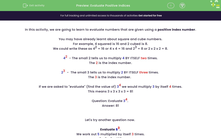# Evaluate Positive Indices

In this worksheet, students will find the value of numbers when positive and negative integer indices (or powers) are used.Key stage:  KS 4

Year:  GCSE

GCSE Subjects:   Maths

GCSE Boards:   Pearson Edexcel, OCR, Eduqas, AQA,

Curriculum topic:   Number, Indices and Surds

Curriculum subtopic:   Structure and Calculation Powers and Roots

Difficulty level:#### Worksheet Overview

In this activity, we are going to learn to evaluate numbers that are given using a positive index number.

For example, 4 squared is 16 and 2 cubed is 8.

We could write these as 42 = 16 or 4 x 4 = 16 and 23 = 8 or 2 x 2 x 2 = 8.

42  - The small 2 tells us to multiply 4 BY ITSELF two times.

The is the index number.

2 -  The small 3 tells us to multiply 2 BY ITSELF three times.

The is the index number.

If we are asked to "evaluate" (find the value of) 34 we would multiply by itself times.

This means 3 x 3 x 3 x 3 = 81

Question: Evaluate 34

Let's try another question now.

Evaluate 53.

We work out multiplied by itself times.

5 x 5 x 5 = 125

We are now going to work out (-5)3.

We will need to multiply (-5) by itself 3 times and we will need to follow the rules for multiplying negative numbers

This means we need to calculate: (-5) x (-5) x (-5)

The first step is to find (-5) x (-5)

Two negative numbers multiply to make a positive number, so the answer to this step is +25.

The next step is to multiply this result by (-5).

25 x (-5) = -125

In this activity, we will evaluate positive and negative indices to work out the whole number value of each and whether the outcome is positive or negative.

You will need to have your scientific calculator handy for some of the questions in this activity.

### What is EdPlace?

We're your National Curriculum aligned online education content provider helping each child succeed in English, maths and science from year 1 to GCSE. With an EdPlace account you’ll be able to track and measure progress, helping each child achieve their best. We build confidence and attainment by personalising each child’s learning at a level that suits them.

Get started